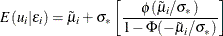The QLIM Procedure

Output to SAS Data Set

Subsections:

XBeta, Predicted, Residual

Xbeta is the structural part on the right-hand side of the model. Predicted value is the predicted dependent variable value. For censored variables, if the predicted value is outside the boundaries, it is reported as the closest boundary. For discrete variables, it is the level whose boundaries Xbeta falls between. Residual is defined only for continuous variables and is defined as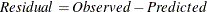Error Standard Deviation

Error standard deviation is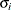in the model. It varies only when the HETERO statement is used.

Marginal Effects

Marginal effect is defined as a contribution of one control variable to the response variable. For the binary choice model with two response categories,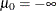,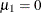,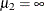; and ordinal response model with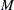response categories,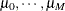, define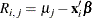The probability that the unobserved dependent variable is contained in the jth category can be written as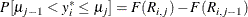The marginal effect of changes in the regressors on the probability of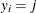is then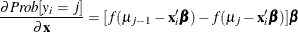where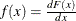. In particular,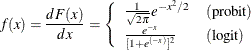The marginal effects in the Box-Cox regression model are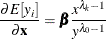The marginal effects in the truncated regression model are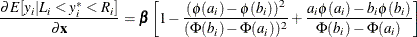where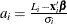and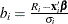.

The marginal effects in the censored regression model are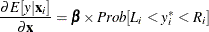Inverse Mills Ratio, Expected and Conditionally Expected Values

Expected and conditionally expected values are computed only for continuous variables. The inverse Mills ratio is computed for censored or truncated continuous, binary discrete, and selection endogenous variables.

Let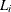and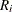be the lower boundary and upper boundary, respectively, for the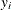. Defineand. Then the inverse Mills ratio is defined as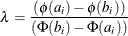for a continuous variable and defined as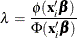for a binary discrete variable.

The expected value is the unconditional expectation of the dependent variable. For a censored variable, it is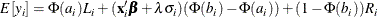For a left-censored variable (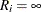), this formula is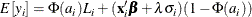where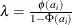.

For a right-censored variable (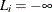), this formula is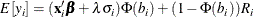where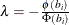.

For a noncensored variable, this formula is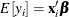The conditional expected value is the expectation given that the variable is inside the boundaries: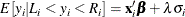Probability

Probability applies only to discrete responses. It is the marginal probability that the discrete response is taking the value of the observation. If the PROBALL option is specified, then the probability for all of the possible responses of the discrete variables is computed.

Technical Efficiency

Technical efficiency for each producer is computed only for stochastic frontier models.

In general, the stochastic production frontier can be written as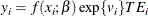wheredenotes producer i’s actual output,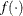is the deterministic part of production frontier,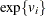is a producer-specific error term, and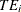is the technical efficiency coefficient, which can be written as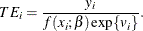In the case of a Cobb-Douglas production function,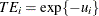. See the section Stochastic Frontier Production and Cost Models.

Cost frontier can be written in general as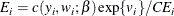where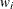denotes producer i’s input prices,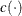is the deterministic part of cost frontier,is a producer-specific error term, and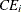is the cost efficiency coefficient, which can be written as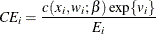In the case of a Cobb-Douglas cost function,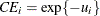. See the section Stochastic Frontier Production and Cost Models. Hence, both technical and cost efficiency coefficients are the same. The estimates of technical efficiency are provided in the following subsections.

Normal-Half Normal Model

Define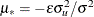and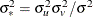. Then, as it is shown by Jondrow et al. (1982), conditional density is as follows: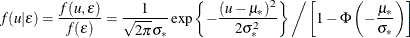Hence,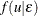is the density for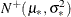.

Using this result, it follows that the estimate of technical efficiency (Battese and Coelli, 1988) is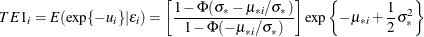The second version of the estimate (Jondrow et al., 1982) is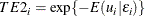where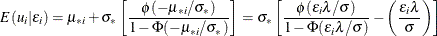Normal-Exponential Model

Define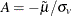and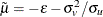. Then, as it is shown by Kumbhakar and Lovell (2000), conditional density is as follows: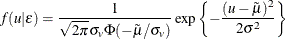Hence,is the density for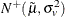.

Using this result, it follows that the estimate of technical efficiency is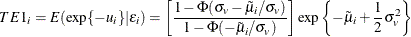The second version of the estimate iswhere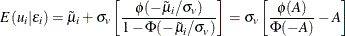Normal-Truncated Normal Model

Define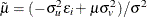and. Then, as it is shown by Kumbhakar and Lovell (2000), conditional density is as follows: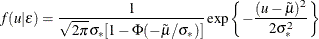Hence,is the density for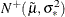.

Using this result, it follows that the estimate of technical efficiency is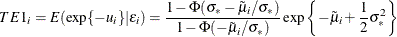The second version of the estimate iswhere